# 计算机视觉实战(八）直方图与傅里叶变换

## 直方图直观理解

我们都知道图像是由像素点组成的，这一节我们就来统计这个图像中的像素点。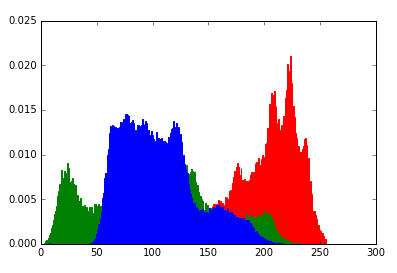它的横坐标表示像素点，纵坐标表示出现的频率。也就是统计图像中某个像素点出现的频率。依据这个定义，这个像素点的范围就是0-255

## calcHist()函数解释

我们现在来看一下在PythonOpenCv库中的统计直方图的函数。

cv2.calcHist([img],,None,,[0,256])


其函数及其参数解释如下：

cv2.calcHist(images,channels,mask,histSize,ranges)

• images: 原图像图像格式为 uint8 或 ﬂoat32。当传入函数时应 用中括号 [] 括来例如[img]

• channels: 同样用中括号括来它会告函数我们统幅图像的直方图。如果入图像是灰度图它的值就是 如果是彩色图像 的传入的参数可以是  它们分别对应着 BGR。相当于统计不同的通道的直方图。

• mask: 掩模图像。统整幅图像的直方图就把它为 None。但是如果你想统图像局部的直方图的你就得制作一个掩模图像，并将它作用在这个图像上，就会统计掩模这部分的直方图。

• histSize:BIN 的数目。表示的是你需要统计的像素范围。你也可以选择[0-10]范围的像素频率统计。

• ranges: 像素值范围常为 。

这个函数的返回值是一个二维的，第一个维度是像素点的范围，第二个维度是像素点的频率。

## 代码实战

我们以lena图像为例进行直方图统计。先看一下图像长啥样。

def cv_show(img,name):
cv2.imshow(name,img)
cv2.waitKey()
cv2.destroyAllWindows()

img = cv2.imread('lena.jpg',0) #0表示灰度图
cv_show(img,'lena')


结果显示如下图所示：对其进行直方图统计，结果显示如下所示：

hist = cv2.calcHist([img],,None,,[0,256]) # 统计直方图
plt.hist(img.ravel(),256)
plt.show() # 显示图像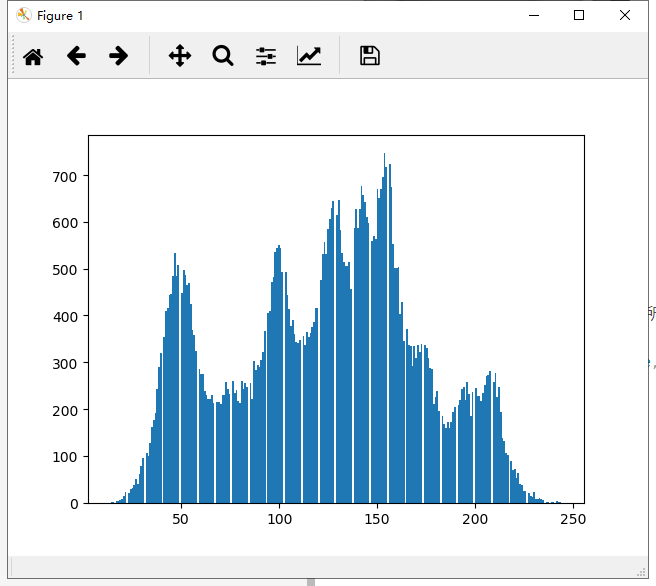### Mask后直方图统计

我们需要先做一个掩码，

## 做一个掩码
mask = np.zeros(img.shape[:2], np.uint8)
print (mask.shape)
mask[100:300, 100:400] = 255


之后我们可以先试一下这个掩码，就是看一下掩码与原图像与一下，之后就能区分被掩码操作了的部分。我们再统计掩码过后跟原图像的直方图。我们再画图将其显示。

## 显示一下这个掩码
masked_img = cv2.bitwise_and(img, img, mask=mask)#与操作
# cv_show(masked_img,'masked_img')

## 统计原直方图，与mask直方图。
hist_full = cv2.calcHist([img], , None, , [0, 256])
hist_mask = cv2.calcHist([img], , mask, , [0, 256])

## 画图
plt.subplot(221), plt.imshow(img, 'gray')
plt.subplot(222), plt.imshow(mask, 'gray')
plt.subplot(223), plt.imshow(masked_img, 'gray')
plt.subplot(224), plt.plot(hist_full), plt.plot(hist_mask)
plt.xlim([0, 256]) # 设置x轴的数值显示范围。
plt.show()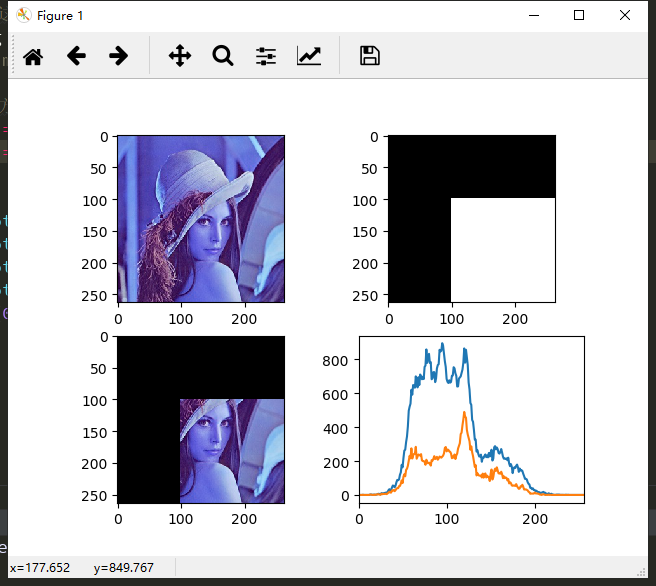## 直方图均衡化

从上面结果我们可以看出，这个直方图有些地方非常密集，有些地方像素点的频率比较低。可以用直方图均衡化处理一下。均衡化原理这里就不详细介绍了，可以上网多查看点文章吧。

调用OpenCV中的equalizeHist可以将其均衡化。

import cv2 #opencv读取的格式是BGR
import matplotlib.pyplot as plt#Matplotlib是RGB

def cv_show(img,name):
cv2.imshow(name,img)
cv2.waitKey()
cv2.destroyAllWindows()

img = cv2.imread('lena.jpg',0) #0表示灰度图 #clahe

equ = cv2.equalizeHist(img)
plt.subplot(121)
plt.hist(img.ravel(),256) # 均衡化前

plt.subplot(122)
plt.hist(equ.ravel(),256) # 均衡化后

plt.show()


结果显示如下所示：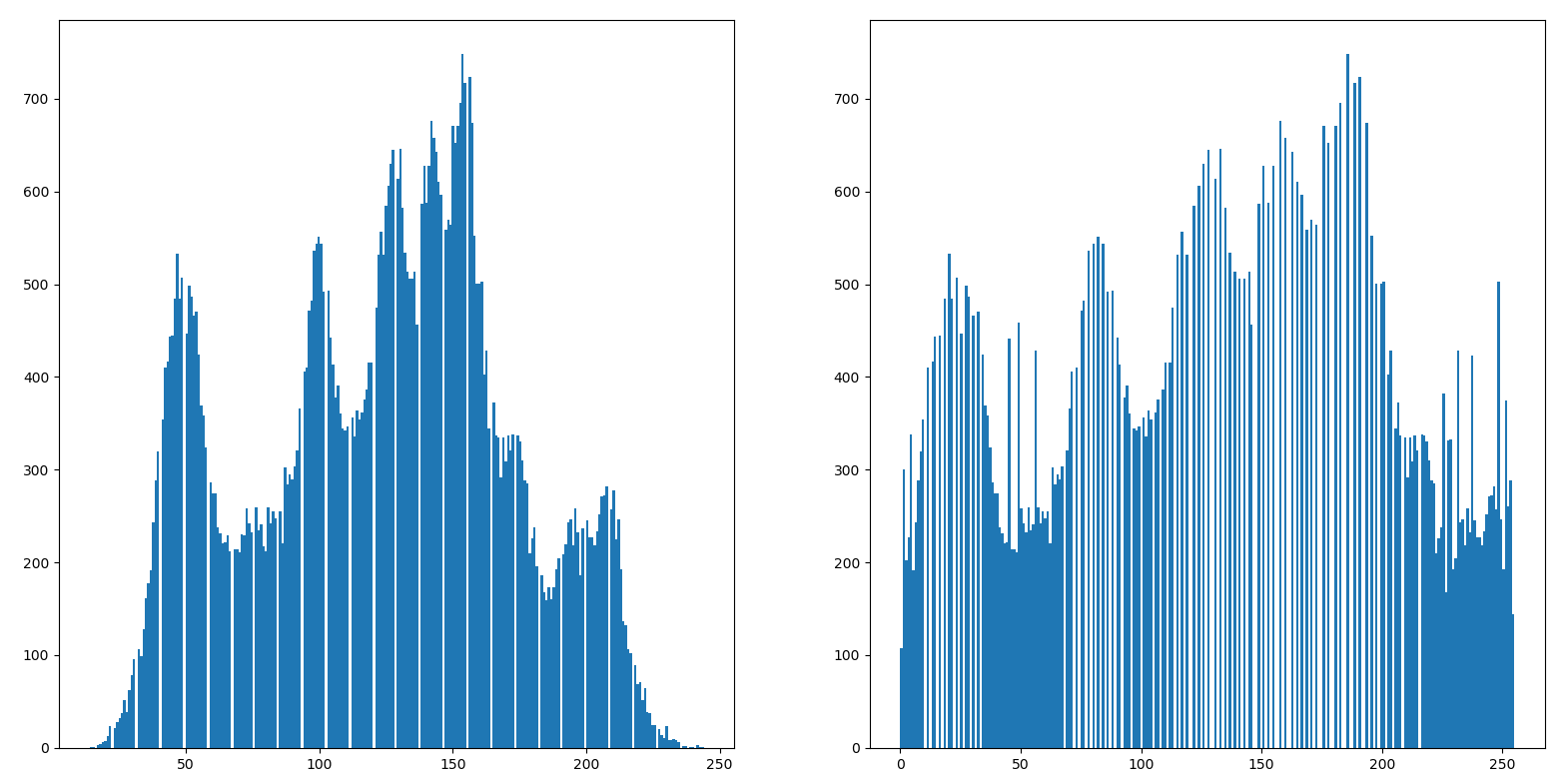## 自适应均衡化

如果我们对局部进行均衡化，而不是全局均衡化的话，图像能保留局部纹理信息。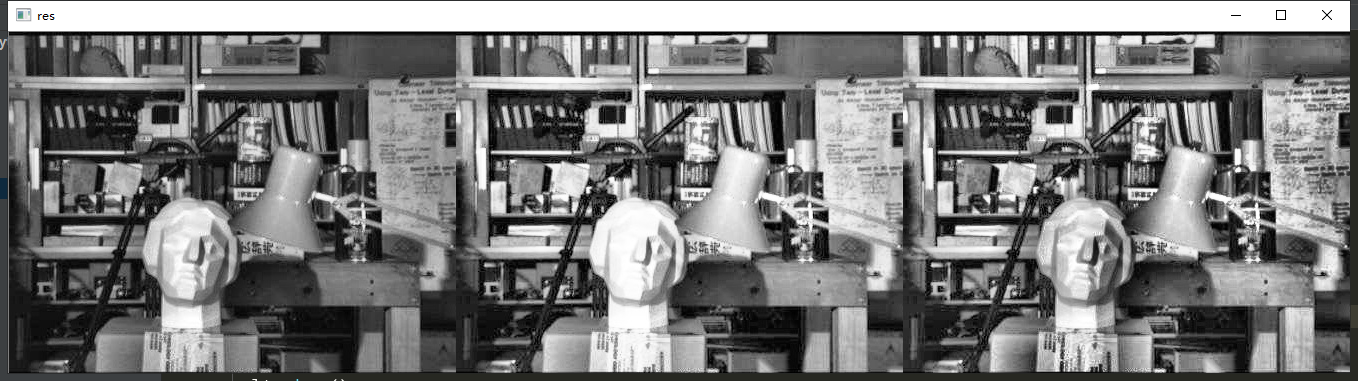从上述结果可以看出，均衡化之后会变更亮一点，自适应均衡化能够保留人脸的纹理信息。

## 傅里叶变换

我们生活中大多数时候是随时间变化的。而频域里面，主要是统计你做事情的规律。感兴趣的可以阅读这篇文章：https://zhuanlan.zhihu.com/p/19763358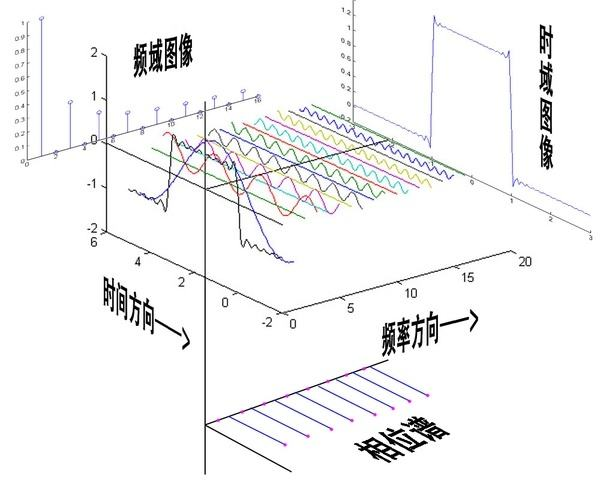• opencv中主要就是cv2.dft()cv2.idft()两个函数对图像进行变换，输入图像需要先转换成np.float32 格式。

• 得到的结果中频率为0的部分会在左上角，通常要转换到中心位置，可以通过shift变换来实现。

• cv2.dft()返回的结果是双通道的（实部，虚部），通常还需要转换成图像格式才能展示(0,255)

获取频谱图并显示：

import numpy as np
import cv2
from matplotlib import pyplot as plt

img = cv2.imread('lena.jpg',0)

img_float32 = np.float32(img)

dft = cv2.dft(img_float32, flags = cv2.DFT_COMPLEX_OUTPUT)
dft_shift = np.fft.fftshift(dft) # np中shift变换，将其转到中心
# 得到灰度图能表示的形式
magnitude_spectrum = 20*np.log(cv2.magnitude(dft_shift[:,:,0],dft_shift[:,:,1]))

plt.subplot(121),plt.imshow(img, cmap = 'gray')
plt.title('Input Image'), plt.xticks([]), plt.yticks([])
plt.subplot(122),plt.imshow(magnitude_spectrum, cmap = 'gray')
plt.title('Magnitude Spectrum'), plt.xticks([]), plt.yticks([])
plt.show()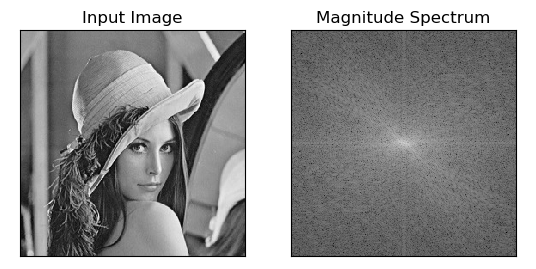其中心位置是低频部分，我们我们只要低频部分的话，在中心位置做一个掩码就可以获得了。之后再进行逆变换即可获得。

import numpy as np
import cv2
from matplotlib import pyplot as plt
img = cv2.imread('lena.jpg',0)

img_float32 = np.float32(img)

dft = cv2.dft(img_float32, flags = cv2.DFT_COMPLEX_OUTPUT)
dft_shift = np.fft.fftshift(dft)

rows, cols = img.shape
crow, ccol = int(rows/2) , int(cols/2)     # 中心位置

# 高通滤波
mask = np.ones((rows, cols, 2), np.uint8)
mask[crow-30:crow+30, ccol-30:ccol+30] = 0

# IDFT
fshift = dft_shift*mask
f_ishift = np.fft.ifftshift(fshift)
img_back = cv2.idft(f_ishift)
img_back = cv2.magnitude(img_back[:,:,0],img_back[:,:,1])

plt.subplot(121),plt.imshow(img, cmap = 'gray')
plt.title('Input Image'), plt.xticks([]), plt.yticks([])
plt.subplot(122),plt.imshow(img_back, cmap = 'gray')
plt.title('Result'), plt.xticks([]), plt.yticks([])

plt.show()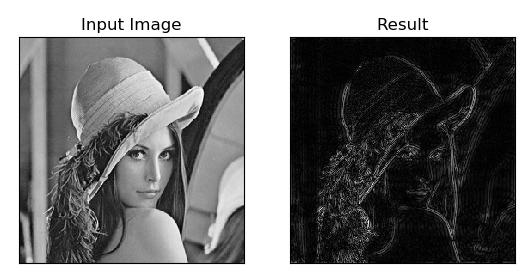12-2802-21615
05-071083
12-1455
05-0768
04-27552
04-2810
03-23152
09-19372
02-203228
09-2776
12-1211
01-25420
12-1828
10-221525
04-064346
©️2020 CSDN 皮肤主题: 猿与汪的秘密 设计师:白松林